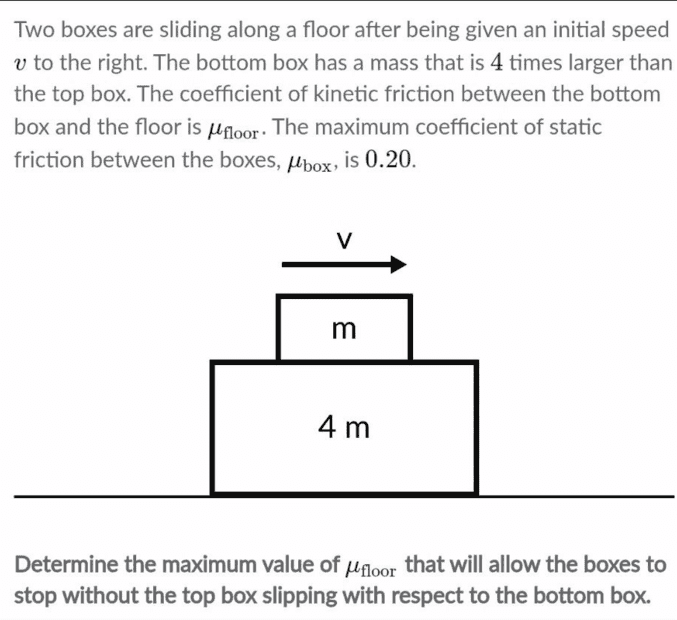# Help Me Solve This Khan Academy Question: Friction and Initial Velocity

• Iwanttolearnphysics
In the first situation, the box would move at a constant speed and the acceleration would be zero.In the second situation, the box would move at a constant speed and the acceleration would be the same as the initial velocity.f

#### Iwanttolearnphysics

Homework Statement
Two boxes are sliding along a floor after being given an initial speed v to the right. The bottom box has a mass that is 4x larger than the top box. The coefficient of kinetic friction between the bottom box and the floor is μfloor. The maximum coefficient of static friction between the boxes, μbox is 0.20. Determine the maximum value of μfloor, that will allow the boxes to stop without the top box slipping with respect to the bottom box.
Relevant Equations
Ff = Fn x μ
Here's a picture of the question:This is a Khan Academy question and although I could just click on hint to find out what the answer is, I think it would be helpful to still ask this here before looking at the answer over there, so that I know what I did wrong. Thank you in advance to anyone willing to help me.

Here's my thinking:
• Ff = Fn x μbox
• Ff = mg x μbox
• Ff = 1(9.81) x 0.20
• Ff = 1.96 N
• That's the friction between box m and box 4m.
• I don't know how that would help me solve the problem. It says to determine the maximum value of μfloor. How do I even relate them to each other?
• I can use Ff = Fn x μfloor
• Ff = mg x μbox
• Ff = 5(9.81) x μbox
• Now I'm stuck. I can't assume that the Friction for the floor and box m are the same as what I computed before.
• I don't know what I'm missing. It says the box was given an initial velocity, "v". But how is that helpful?
I do not like problems without numbers. I cannot make sense of them. This tells me that there's still so much I don't understand.

It says the box was given an initial velocity, "v". But how is that helpful?
What matters is that it is moving. If the top box is not slipping, what can you say about the accelerations? Relate those to the forces.

•Iwanttolearnphysics
What matters is that it is moving. If the top box is not slipping, what can you say about the accelerations? Relate those to the forces.
I thought about that too, but I think I made a mistake so I scrapped that idea. I thought about acceleration possibly being zero because the boxes are in contact with each other and the top one is not slipping, my equation became:
• Ff = Fn x μfloor
• ma = Fn x μfloor
• 5(0) = 5(9.81) x μfloor
• μfloor = -49.05?
• I do not think this is right at all. μ is usually a very small number and not negative.
• Also, in the equation I wrote above, it's right that I added the masses right? Since the top box is adding to the weight force of the bottom one (thus, affecting it's normal force).
• I also was not able to use the 0.20 in this equation. I don't know how to relate these two things to each other.
Thank you very much.

I thought about acceleration possibly being zero because the boxes are in contact with each other and the top one is not slipping
Which acceleration? The boxes are not going to slide forever on a frictional floor.

Which acceleration? The boxes are not going to slide forever on a frictional floor.
You're right. Let me think about this problem some more. I am stuck and although what you said makes sense, I cannot seem to apply it to this problem. Thank you for your help! I'll figure it out and come back here.

Think about how the boxes would behave in each of both following extreme situations:
1) Zero coefficient of friction between both boxes, but some friction between the lower box and the floor.
1) Some friction between both boxes, but zero coefficient of friction between the lower box and the floor.

•Iwanttolearnphysics